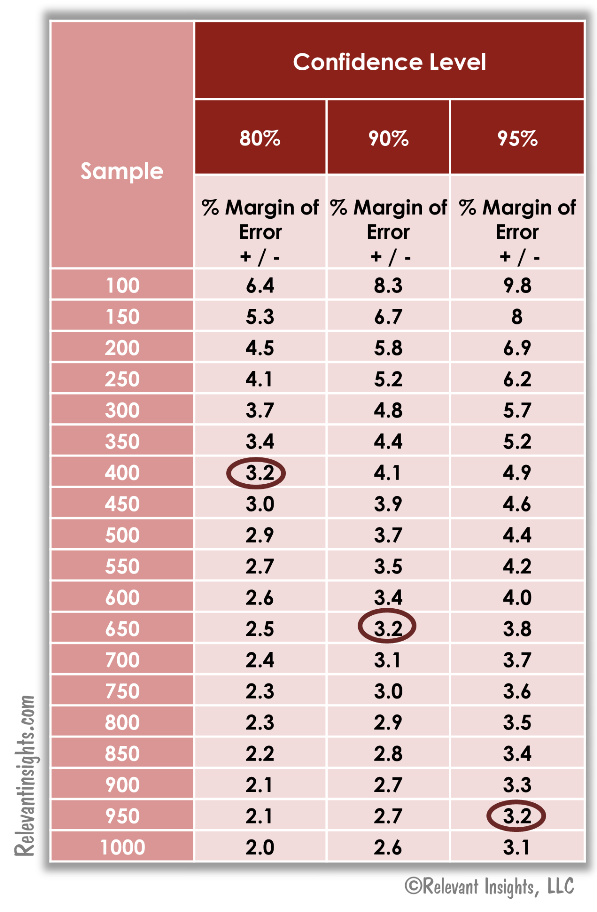# What Is The Right Sample Size For A Survey?

|  Posted: by

Determining the sample size is one of the early steps that must be taken in the planning of a survey. Unfortunately, there is no magic formula for the perfect sample size. In other words, there are several factors we need to think about.

## Analytical Plan

The research objectives and planned analytical approach should be the first factor to consider when making the decision on sample size. For instance, there are statistical procedures (e.g. regression analysis) that require a certain number of observations per variable.

Moreover, if we want to do a comparative analysis between subgroups, we should adjust the sample size for it to identify statistically significant differences between the groups.

## Population Variability

This refers to the target population’s diversity. If the target population exhibits large variability (i.e., standard deviation) in the behaviors and attitudes being researched, a large sample is needed. If 20% or 80% of the population behaves in a certain way, this indicates less variability than if 50% would do so.

To be conservative, it is standard practice to use 50% (0.5) as the event probability in sample size calculations since it represents the highest variability that can be expected in the population.

## Level of Confidence

This is the level of risk we are willing to tolerate usually expressed as a percentage (e.g., 95% confidence level). Although survey results are reported as point estimates (e.g., 75% of respondents like this product), we can only be confident that our method indicates that the true value of the estimate falls within a particular range or what is called a confidence interval. The reason behind this is that we are working with a sample of the target population, not the total population.

The level of confidence indicates the probability that the true value of the estimate, in fact, will fall within the boundaries of the confidence interval.

How confident can you be in your estimation method? As confident as your tolerance for risk allows you to, knowing that the confidence level is inversely proportional to estimate accuracy or margin of error.

The more confident you want to be, the larger the confidence interval has to be. In turn, this leads to lower levels of precision.

## Margin of Error

Also known as sampling error, indicates the desired level of precision of the estimate. You have probably seen poll results quoted in the media, saying that the margin of error was plus or minus a particular percentage (e.g. +/-3%). This percentage defines the lower and upper bounds of the confidence interval likely to include the parameter estimate, and it is a measure of its reliability. The larger the sample, the smaller the margin of error and the greater the estimate precision.

Below is a table illustrating how the margin of error and level of confidence interact with sample size. To get the same level of precision (e.g. +/-3.2%), we need larger samples as the confidence level increases.

For example, if we want to be certain that in 95 out of 100 times we do the survey the estimate will be +/- 3.2%, we need a sample of 950.For more help on calculating sample size and margin of error, use our Sample Size and Margin of Error Calculators.

## Sample Cost

Sample size cost is often one of the largest items in the budget for market research studies, especially if the target sample includes low-incidence segments or the response rates are low. Many times, our clients have to make a tradeoff between statistical accuracy and research cost.

Recently, I received a call from a client who wanted to conduct an online survey with a sample of 1,000 respondents, which would give a statistical accuracy of +/-3.1% at the 95% confidence level, but would cost \$8,000 based on certain screening criteria.

At the same time, a sample of 400 respondents would give a statistical accuracy of +/-4.9% and cost \$3,400. In this case, a 135% increase in sample cost would only yield a 60% gain in statistical accuracy. The client decided to conduct the study on the smaller sample.

## Population Size

Most of the time, the size of the total target population is unknown, and it is assumed to be large ( >100,000), but in studies where the sample is a large fraction of the population of interest, some adjustments may be needed.

## Sample Size Calculation Check List

As a summary, to determine the sample size needed in a survey, we need to answer the following questions:

• What type of data analysis will be conducted? Will subgroups be compared?
• What is the probability of the event occurring? – If not previous data exists, use 50% for a conservative sample size estimate.
• How much error is tolerable (confidence interval)? What level of precision do we need (margin of error)?
• How confident do we need to be in the method used (confidence level) to determine that the true value falls within the confidence interval?
• What is the research budget? Can we afford the desired sample?
• How large is the population size? Large? Small/Finite? If unknown, assume it to be large ( >100,000).

So, the answer to the question “What is the right sample size for a survey?” is: It depends. I hope I gave you some guidance in choosing sample sizes, but the final decision is up to you. To calculate sample size and margin of error, use our Sample Size and Margin of Error Calculators.

Have you wondered, what sample size is needed to get a representative sample, read Does A Large Sample Size Guarantee A Representative Sample?

(An earlier version of this article was originally published on May 6, 2010. The article was last updated and revised on August 12, 2019.)

Only logged in users can leave comments.